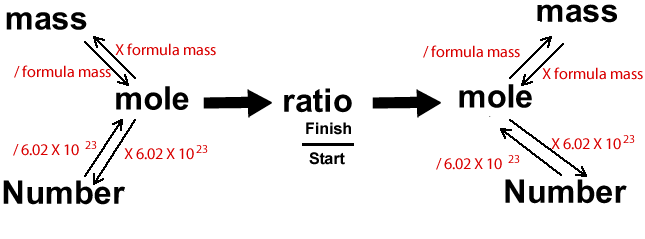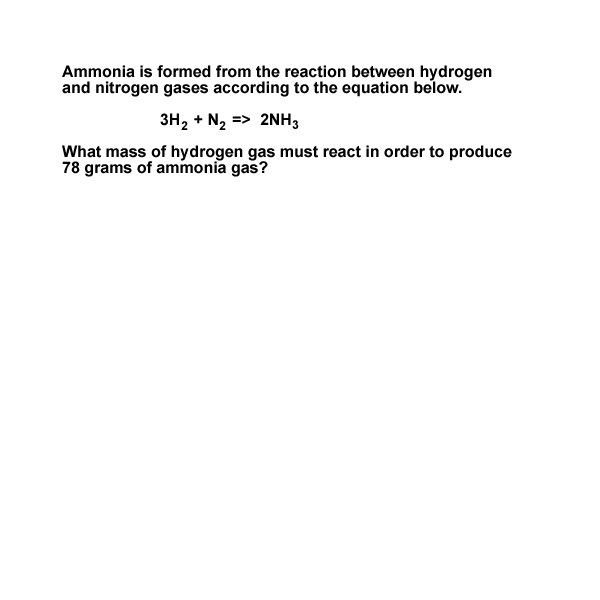Calculations through the stoichiometry diagram Calculations in chemistry can be a little overwhelming. It can sometimes help to visualise the problem through diagrams. Below is a diagram that I find very useful indeed.Lets see how it works. Below is a typical question you may expect to have to answer in stoichiometry. Follow the animation to see how to use the diagram effectively. Hydrogen reacts with nitrogen to produce ammonia according to the equation below. 3 H2 + N2 => 2NH3 W hat is the mass of hydrogen that must react in order to produce 78 grams of ammonia? We are asked for the mass of ammonia and we are given the mass of hydrogen gas that reacts. We must therefore start at "mass", on the right of the diagram, because we know the mass of ammonia and go to "mass", on the other side of the diagram, to find the mass of hydrogen.Continue